# Cyclist

Cyclist goes uphill 10 km for 50 minutes and downhill minutes for 29 minutes, both applied to the pedals same force. How long he pass 10 km by plane?

Correct result:

t =  36.7 min

#### Solution: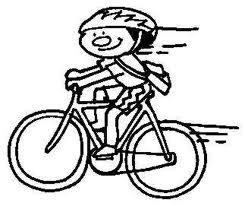We would be very happy if you find an error in the example, spelling mistakes, or inaccuracies, and please send it to us. We thank you!Tips to related online calculators
Looking for calculator of harmonic mean?
Looking for a statistical calculator?
Need help calculate sum, simplify or multiply fractions? Try our fraction calculator.
Check out our ratio calculator.

## Next similar math problems:In six baskets, the seller has fruit. In individual baskets, there are only apples or just pears with the following number of fruits: 5,6,12,14,23 and 29. "If I sell this basket," the salesman thinks, "then I will have just as many apples as a pear." Whic
• TractorsFields go plow two tractors with various performance. The first tractor plow whole field in 11 hours, the second tractor plow whole field 19 hours longer. How long take plow whole field with two tractors?
• Final exam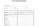At the final exam, the student answers from three areas, which are evaluated in a ratio of 1: 2: 2. What grade will John receive if he answered as follows: 3,1,2.
• BW-BS balls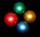Adam has a full box of balls that are large or small, black or white. Ratio of large and small balls is 5:3. Within the large balls the ratio of the black to white is 1:2 and between small balls the ratio of the black to white is 1:8 What is the ratio of
• Three numbers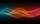The sum of the three numbers is 287. These numbers are in a ratio of 3: 7: 1/4 Define these numbers
• Copiers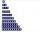Three copiers will do the same in an hour k copies. How many copies would be done in an hour by four such copiers? ?
• The angles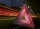The angles in the triangle are in the ratio 12: 15: 9. Find the angles.
• Red diplomasHe numbers of students with honors in 2013 and 2014 are in ratio 40:49. How big is the year-on-year percentage increase?
• The diceWhat is the probability of events that if we throw a dice is rolled less than 6?
• Cake ingredients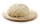The yeast cake contains milk, yeast, sugar, and flour in a ratio of 20: 4: 1: 15. What percentage of flour is in yeast?
• Fast tourists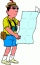If three tourists pass the route in 5 hours, how long will the same route take six equally fast tourists?
• Three numbers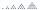What is three numbers which have the property: the sum of the reciprocals of the first and second numbers is 12/7, the first and third 11/24 and the second and the third 3/8.
• Numbers at ratio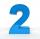The two numbers are in a ratio 3:2. If we each increase by 5 would be at a ratio of 4:3. What is the sum of original numbers?
• Eq with reciprocalSolve given equation with reciprocal member: a-6/a+10=4/8
• On a farm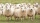On a farm, there are 90 rabbits, 700 sheep, 300 cattle and 500 pigs. What percentage of the total number of animals are rabbits?
• Land areaA land area of Asia and Africa are in a 3: 2 ratio, the European and African are is 1:3. What are the proportions of Asia, Africa, and Europe?
• CandiesIf Alena give Lenka 3 candy will still have 1 more candy. If Lenka give Alena 1 candy Alena will hame twice more than Lenka. How many candies have each of them?